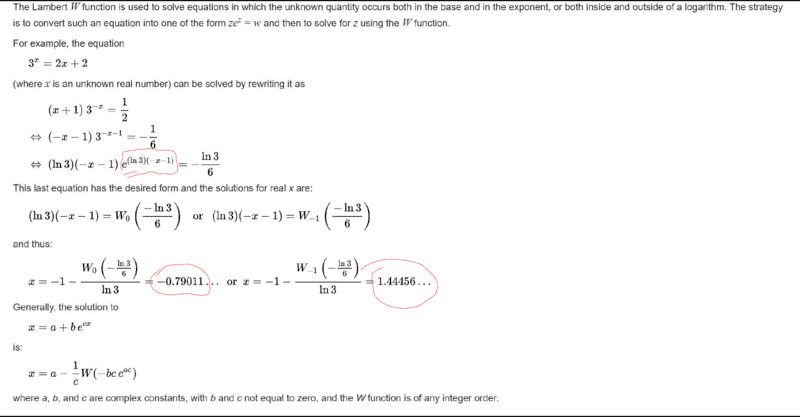# Solve the given equation using the Lambert W function

• chwala

#### chwala

Gold Member
Homework Statement
Solve for ##x## given ##3^x=2x+2##
Relevant Equations
Lambert W Function
I just came across this...the beginning steps are pretty easy to follow...i need help on the highlighted part as indicated below;From my own understanding, allow me to create my own question for insight purposes...

let us have;

##7^x=5x+5##

##\dfrac{1}{5}=(x+1)7^{-x}##

##\dfrac{1}{35}=(x+1)7^{(-x-1)}## this is clear...
then we desire our equation to be in the form;
##we^w##
then we shall have,

##-\dfrac{1}{35}=(-x-1)7^{(-x-1)}##

Let ##y=7^{(-x-1)}##

then ##\ln y=(-x-1)\ln 7##

##⇒e^{(-x-1)\ln 7}=y## then on substituting back on ##-\dfrac{1}{35}=(-x-1)7^{(-x-1)}## and multiplying both sides of the equation by ##\ln 7##

we get;

##(\ln 7)(-x-1)⋅ e^{(-x-1)\ln 7} = \dfrac {-\ln 7}{35}##

##(\ln 7)(-x-1)=W_0 \left[\frac{-\ln 7}{35}\right ]##

or
##(\ln 7)(-x-1)=W_{-1} \left[\frac{-\ln 7}{35}\right ]## how do we arrive at the required values from here? Is there a table?

Last edited:
The values of Lambert W are numerically evaluated. I believe Newton's method should be a solid pick and wikipedia recommends it, as well. Otherwise, it looks like you have correctly followed the guide. I wouldn't worry too much about numerical values unless you needed to implement them.

•jim mcnamara
I hear you. Thanks for your feedback. I may explore this in detail later on how they arrived at the numerical values. Newton's method handles this quite well.